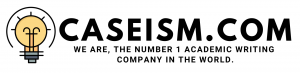# USEC INC. Case Solution & Answer

Analysis

USEC pursuing ACP

With the arrival of maturity period of the power supply contract of USEC the costs of enrichment process would increase and this will lead to higher costs for the company, hence reducing profitability and the competitive advantage. In order to avoid this, the company is pursuing an alternative option that could help in maintaining the competitive advantage of the company and improve its profitability.

The alternative which the company is considering is American Centrifuge Project (ACP) as the most potential project for their objective. The fruitful prospects of ACP contributed to the driving force for the company to pursue this projects

USEC INC. Case Solution & Answer

Despite the massive investment and high initial costs the project seemed to be very fertile in terms of cost savings and productive capability. Firstly, the reduction in per unit cost of Separative Work Unit (SWU) to about fifty percent of the existing costs, for which the company finds ACP in line with increasing the profitability as well as the capability of the company in its industry.

ACP is a cheaper and more efficient project for enriching uranium which have prospects of massive savings from this new project as well as will seek to maintain productivity of the company pertaining to the demand.

Apart from this, the company will able to save costs of electricity used in enriching uranium by 5% while reducing the overall costs by 50%. The production capability of the new project would start from 2.5 million SWU which will rise up to 6.5 million SWU in 2013 which determines the adaptability of the project in a short time period.

Weighted Average Cost of Capital (WACC)

Weighted Average Cost of Capital (WACC) is calculated as shown in the excel sheet. The WACC calculated is based on assumptions which are mentioned in the data column which depicts the assumptions as well as the basis for these assumptions.

Firstly, the cost of debt is taken as the yield to maturity of the debts of USEC, market premium as average of the market rate of return, risk free rate as 30 year Treasury bond rate, tax rate of 40% as given in the case and finally the cost of equity is calculated using this data and the formula for cost of equity as shown.

The weighted average cost of capital is then calculated to be 9.9% for analyzing the new and existing projects of USEC. This has been calculated through the formula as shown in excel. However, this discount rate is questionable as the assumptions taken might not reflect the actual situation of the project and its factors that could affect the percentage. Therefore, in order to make it more accurate more measures needed to be incorporated. Nevertheless, this percentage can be used as a good starting point for the evaluation of the new project.

Estimating NPV and IRR of ACP

In order to estimate the IRR and NPV of the new project, ACP some further assumptions are taken for estimating the forecasts of revenues and costs. Such as, the inflation rate over the time period is estimated to be 3% as determined by Mackovjak after the discussion with Dr. Peter. The process for SWU and uranium to be \$127 and \$43 respectively, and are increasing at the assumed rate of inflations.

After formulating the revenues and costs of the operations under the two projects Paducah and ACP the profits before tax and after tax for the year are calculated which are then discounted at the cost of capital calculated before…………………..

This is just a sample partial work. Please place the order on the website to get your own originally done case solution